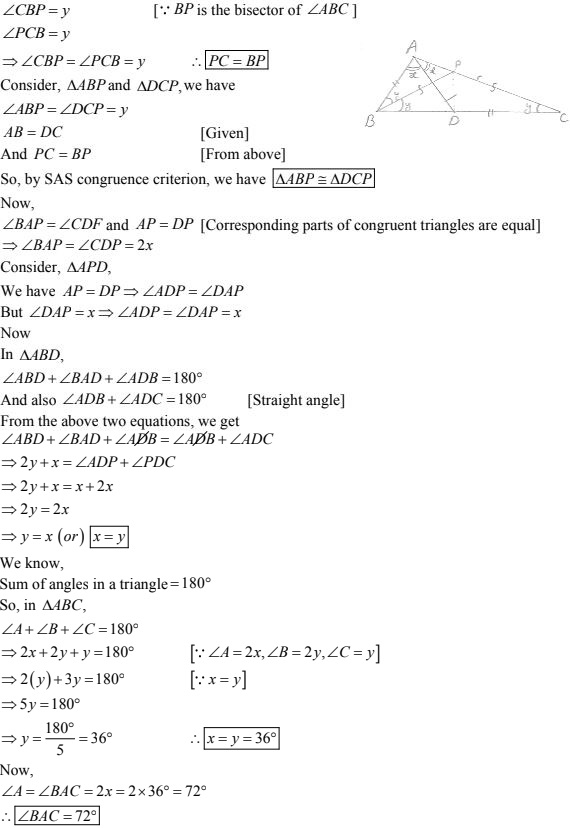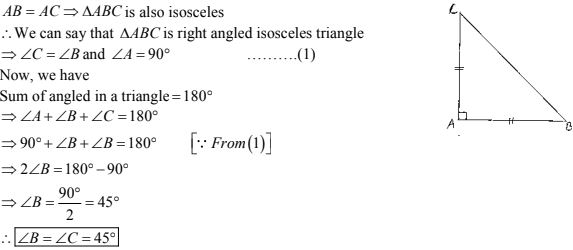# Chapter 10 Congruent Triangles RD Sharma Solutions Exercise 10.3 Class 9 MathsChapter Name RD Sharma Chapter 10 Congruent Triangles Exercise 10.3 Book Name RD Sharma Mathematics for Class 10 Other Exercises Exercise 10.1Exercise 10.2Exercise 10.3Exercise 10.5Exercise 10.6 Related Study NCERT Solutions for Class 10 Maths

### Exercise 10.3 Solutions

1. In two right triangles one side an acute angle of one are equal to the corresponding side and angle of the other. Prove that the triangles are congruent.

Solution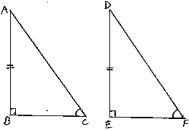Given that, in two right triangles one side and acute angle of one are equal to the corresponding side and angles of the other.
We have to prove that the triangles are congruent .
Let us consider two right triangles such that
∠B =  ∠C = 90° ...(1)
AB = DE ...(2)
∠C = ∠F ...(3)
Now observe the two triangles ABC and DEF
∠C = ∠F  [From (3)]
∠B = ∠E  [From (1)]
and AB = DE  [From (2)]
So, by AAS congruence criterion, we have ΔABC ≅ ΔDEF
∴ The two triangles are congruent
Hence, proved

2. If the bisector of the exterior vertical angle of a triangle be parallel to the base. Show that the triangle is isosceles.
Solution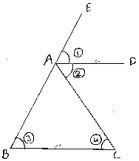Given that the bisector of the exterior vertical angle of a triangle is parallel to the base and we have to prove that the triangle is isosceles
Let ABC be a triangle such that AD is the angular bisector of exterior vertical angle EAC and AD||BC
We have,
and ∠DAC = ∠ACB  [alternative angle]
⇒ ∠ABC = ∠ACB
⇒ AB = AC
Since, in ΔABC, two sides AB and AC are equal we can say that ΔABC is isosceles

3. In an isosceles triangle, if the vertex angle is twice the  sum of the base angles, calculate the angles of the triangle.
Solution
Let ΔABC be isosceles such that AB = AC.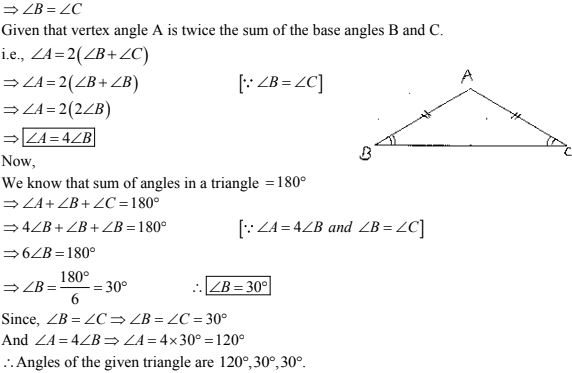4. PQR is a triangle in which PQ  = PR and S is any point on the side PQ. Through S, a line is drawn parallel to QR and intersecting PR at T. Prove that PS = PT.
Solution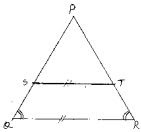Given that PQR is a triangle such that PQ = PR and S is any point on the side PQ and ST || QR.
We have to prove PS = PT
Since, PQ = PR
⇒ ΔPQR is isosceles
⇒ ∠Q = ∠R (or) ∠PQR = ∠PRQ
Now,
∠PST = ∠PQR and ∠PTS = ∠PRQ  [Corresponding angles as ST || QR]
Since, ∠PQR = ∠PRQ
⇒ ∠PST = ∠PTS
Now,
In ΔPST,
∠PST = ∠PTS
⇒ ΔPST is an isosceles triangle
⇒ PS = PT

5. In a ΔABC, it is given that AB = AC and the bisectors of ∠B and ∠C intersect at O. If M is a point on BO produced, prove that ∠MOC = ∠ABC.
Solution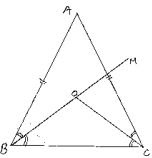Given that in ΔABC,
AB = AC and the bisector ∠B and ∠C intersect at O and M is a point of BO produced,
We have to prove ∠MOC = ∠ABC.
Since,
AB = AC
⇒ ΔABC is isosceles
⇒ ∠B = ∠C (or) ∠ABC = ∠ACB
Now,
BO and CO are bisectors of ∠ABC and ∠ACB respectively.
⇒ ABO = ∠OBC = ∠ACO = ∠OCB = (1/2) (∠ABC) = (1/2) (∠ACB) ...(1)
We have, in ΔOBC
∠OBC + ∠OCB + ∠BOC = 180° ...(2)
And also
∠BOC + ∠COM = 180° ...(3) [Straight angle]
Equating (2) and (3)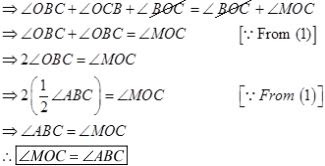6. P is a point on the bisector of an angle ∠ABC. If the line through P parallel to AB meets BC at Q, prove that triangle BPQ is isosceles.
Solution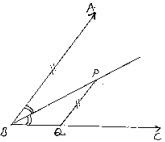Given that P is a point on the bisector of an angle ∠ABC, and PQ || AB.
We have to prove that ΔBPQ is isosceles
Since,
BP is bisector of ∠ABC
⇒ ∠ABP = ∠PBC ...(1)
Now,
PQ || AB
⇒ ∠BPQ = ∠ABP  [alternative angles]
From (1) and (2), we get
∠BPQ = ∠PBC (or) ∠BPQ  = ∠PBQ
Now,
In ΔBPQ,
∠BPQ = ∠PBQ
⇒ ΔBPQ = is an isosceles triangle.
∴ Hence proved

7. Prove that each angle of an equilateral triangle is 60°.
Solution
Given to prove that each angle of an equilateral triangle is 60°.
Let us consider an equilateral triangle ABC
Such that AB = BC = CA
Now,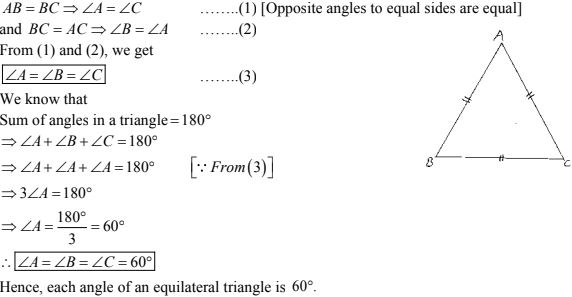8. Angles A, B, C of a triangle ABC are equal to each other. Prove that ΔABC is equilateral.
Solution
Given that angles A, B, C of a triangle ABC equal to each other.
We have to prove that ΔABC is equilateral
We have, ∠A = ∠B = ∠C
Now,
∠A = ∠B
⇒ BC - AC  [Opposite sides to equal angles are equal]
and ∠B = ∠C
⇒ AC = AB
From the above we get
AB = BC = AC
⇒ ΔABC is equilateral

9. ABC is a triangle in which ∠B = 2∠C. D is a point on BC such that AD bisects ∠BAC and AB = CD. Prove that ∠BAC = 72°.
Solution
Given that in ΔABC, ∠B = 2∠C and D is a point on BC such that AD bisectors ∠BAC and AB = CD.
We have to prove that ∠BAC = 72°
Now, draw the angular bisector of ∠ABC, which meets AC in P. join PD
Let C = ∠ACB = y
⇒ ∠B = ∠ABC = 2∠C = 2y and also